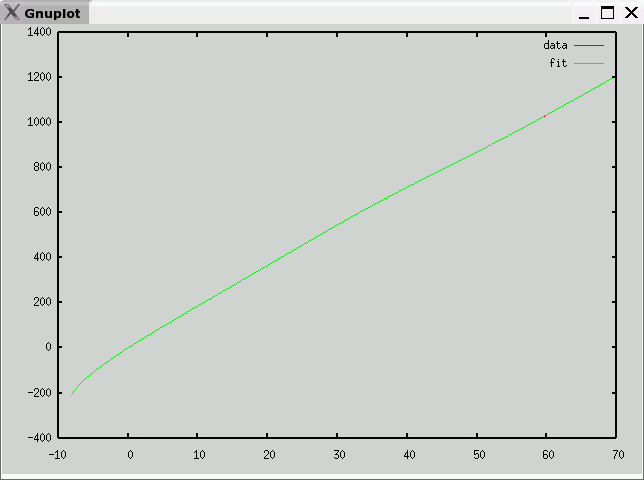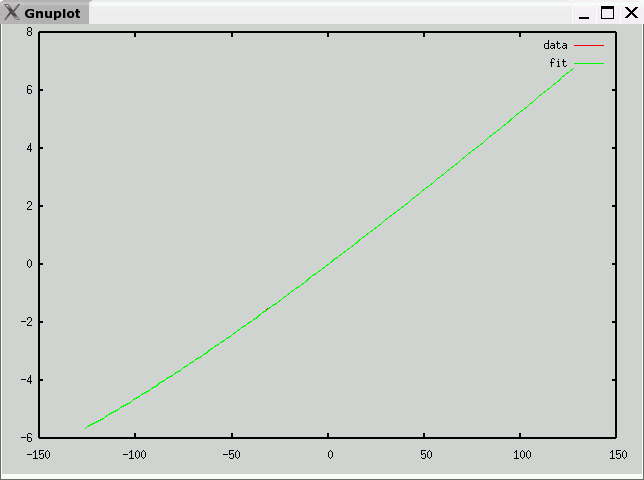# Type J Thermocouple Data and Polynomial

• Description
Fe / Cu-Ni
• Data source
National Institute of Science and Technology (NIST)
• Raw Data
• Octave format
• Curve fit
• mV -> temperature (C)
e is maximum error (in degrees C)
v1 andf v2 partition the domain into three segments, each with a polynomial fit
c is vector of coefficients: a10, a9, ... a0
Note: Each column of c corresponds to one range (<v1,v1 to v2, >v2)

`e = 0.022665v1 = 14.055v2 = 27.057c =   -1.8622e-09    1.0470e-09   4.7507e-13    7.0620e-08   -2.1228e-07  -2.3768e-10   -7.4076e-07    1.9278e-05   5.2834e-08   -1.6808e-06   -1.0326e-03   -6.8649e-06    5.6296e-05   3.6124e-02    5.7683e-04    3.0893e-05   -8.6240e-01  -3.2731e-02   -3.4200e-03    1.4228e+01   1.2699e+00    2.3324e-02   -1.6016e+02  -3.3265e+01   -2.1761e-01    1.1771e+03   5.6321e+02    1.9817e+01   -5.0820e+03  -5.5489e+03   -3.4296e-02    9.8975e+03   2.4405e+04 `• temperature -> mV (range -127C-127C)
e is maximum error (in mV)
c is vector of coefficients: a10, a9, ... a0

`e = 0.00057012c =   -6.9749e-24    1.3658e-21    2.0429e-19   -5.0990e-17   -1.4948e-15    4.6764e-13    1.3473e-10   -8.8655e-08    3.0485e-05    5.0385e-02   -1.0519e-06 `Previous page: Type E
Next page: Type K